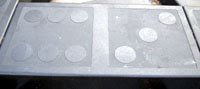## Tuesday, November 18, 2008

### 63

63 = 32 x 7.

63 = 62 + 33 = 26 – 1.

63 is the smallest number such that the common alphabetical value of its representation in Roman numerals is equal to itself: LXIII -- 12 + 24 + 9 + 9 + 9 = 63.

63 is a Harshad (or Niven) number; that is, it is divisible by the sum of its digits.

63 is the number of partially ordered sets of 5 elements.

63 is 333 in base 4.M63 is a galaxy, known as the Sunflower Galaxy, in the constellation Canes Venatici.